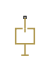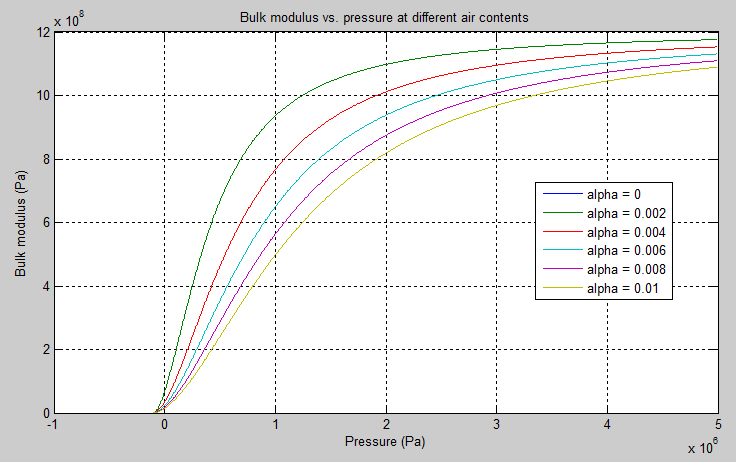# Constant Volume Hydraulic Chamber

Hydraulic capacity of constant volume

## Library

Hydraulic Elements

•## Description

The Constant Volume Hydraulic Chamber block models a fixed-volume chamber with rigid or flexible walls, to be used in hydraulic valves, pumps, manifolds, pipes, hoses, and so on. Use this block in models where you have to account for some form of fluid compressibility. You can select the appropriate representation of fluid compressibility using the block parameters.

Fluid compressibility in its simplest form is simulated according to the following equations:

`${V}_{f}={V}_{c}+\frac{{V}_{c}}{E}p$`

`$q=\frac{{V}_{c}}{E}\cdot \frac{dp}{dt}$`

where

 q Flow rate into the chamber Vf Volume of fluid in the chamber Vc Geometrical chamber volume E Fluid bulk modulus p Gauge pressure of fluid in the chamber

If pressure in the chamber is likely to fall to negative values and approach cavitation limit, the above equations must be enhanced. In this block, it is done by representing the fluid in the chamber as a mixture of liquid and a small amount of entrained, nondissolved gas (see [1, 2]). The mixture bulk modulus is determined as:

`$E={E}_{l}\frac{1+\alpha {\left(\frac{{p}_{a}}{{p}_{a}+p}\right)}^{1/n}}{1+\alpha \frac{{p}_{a}^{1/n}}{n·{\left({p}_{a}+p\right)}^{\frac{n+1}{n}}}{E}_{l}}$`

where

 El Pure liquid bulk modulus pα Atmospheric pressure α Relative gas content at atmospheric pressure, α = VG/VL VG Gas volume at atmospheric pressure VL Volume of liquid n Gas-specific heat ratio

The main objective of representing fluid as a mixture of liquid and gas is to introduce an approximate model of cavitation, which takes place in a chamber if pressure drops below fluid vapor saturation level. As it is seen in the graph below, the bulk modulus of a mixture decreases as the gauge pressure approaches zero, thus considerably slowing down further pressure change. At gauge pressures far above zero, a small amount of undissolved gas has practically no effect on the system behavior.To reproduce this graph, copy and paste the following script in your MATLAB® Command Window:

```% Parameters p_atm = 1.01325e5; % Atmospheric pressure [Pa] pressure = -1.01325e5:1e3:5e6; % Pressure (gauge) [Pa] alpha = 0:2e-3:0.01; % Relative amount of trapped air k_sh = 1.4; % Specific heat ratio bulk = 1.24285e+09; % Bulk modulus at atmospheric pressure and no gas [Pa] % Absolute pressure p_abs = p_atm + pressure; % Relative absolute pressure p_nom = (p_atm./p_abs).^(1/k_sh); p_den = p_nom .* bulk ./ (k_sh .* p_abs); % Instantaneous bulk modulus bulk_inst = bulk * (1+ bsxfun(@times, alpha', p_nom)) ./ (1 + bsxfun(@times, alpha', p_den)); % Reuse figure if it exists, else create new figure if ~exist('h1_bulk_modulus', 'var') || ~isgraphics(h1_bulk_modulus, 'figure') h1_bulk_modulus = figure('Name', 'h1_bulk_modulus'); end figure(h1_bulk_modulus) clf(h1_bulk_modulus) legend_label = cell(length(alpha),1); for i=1:length(alpha) plot(pressure, bulk_inst(i,:)) hold on legend_label{i,1} = ['alpha = ',num2str(alpha(i))]; end grid on xlabel('Pressure (Pa)') ylabel('Bulk modulus (Pa)') title('Bulk modulus vs. pressure at different air contents') legend(legend_label, 'Location', 'Best') hold off```

Cavitation is an inherently thermodynamic process, requiring consideration of multiple-phase fluids, heat transfers, etc., and as such cannot be accurately simulated with Simscape™ software. But the simplified version implemented in the block is good enough to signal if pressure falls below dangerous level, and to prevent computation failure that normally occurs at negative pressures.

If pressure falls below absolute vacuum (–101325 Pa), the simulation stops and an error message is displayed.

If chamber walls have noticeable compliance, the above equations must be further enhanced by representing geometrical chamber volume as a function of pressure:

`${V}_{c}=\pi {d}^{2}/4·L$`

`$d\left(s\right)=\frac{{K}_{p}}{1+\tau s}p\left(s\right)$`

where

 d Internal diameter of the cylindrical chamber L Length of the cylindrical chamber Kp Proportionality coefficient (m/Pa) τ Time constant s Laplace operator

Coefficient `Kp` establishes relationship between pressure and the internal diameter at steady-state conditions. For metal tubes, the coefficient can be computed as (see ):

`${K}_{p}=\frac{d}{{E}_{M}}\left(\frac{{D}^{2}+{d}^{2}}{{D}^{2}-{d}^{2}}+\nu \right)$`

where

 D Pipe external diameter EM Modulus of elasticity (Young's modulus) for the pipe material ν Poisson's ratio for the pipe material

For hoses, the coefficient can be provided by the manufacturer.

The process of expansion and contraction in pipes and especially in hoses is a complex combination of nonlinear elastic and viscoelastic deformations. This process is approximated in the block with the first-order lag, whose time constant is determined empirically (for example, see ).

As a result, by selecting appropriate values, you can implement four different models of fluid compressibility with this block:

• Chamber with rigid walls, no entrained gas in the fluid

• Cylindrical chamber with compliant walls, no entrained gas in the fluid

• Chamber with rigid walls, fluid with entrained gas

• Cylindrical chamber with compliant walls, fluid with entrained gas

The block allows two methods of specifying the chamber size:

• By volume — Use this option for cylindrical or non-cylindrical chambers with rigid walls. You only need to know the volume of the chamber. This chamber type does not account for wall compliance.

• By length and diameter — Use this option for cylindrical chambers with rigid or compliant walls, such as circular pipes or hoses.

The block has one hydraulic conserving port associated with the chamber inlet. The block positive direction is from its port to the reference point. This means that the flow rate is positive if it flows into the chamber.

### Variables

To set the priority and initial target values for the block variables prior to simulation, use the Initial Targets section in the block dialog box or Property Inspector. For more information, see Set Priority and Initial Target for Block Variables.

Nominal values provide a way to specify the expected magnitude of a variable in a model. Using system scaling based on nominal values increases the simulation robustness. Nominal values can come from different sources, one of which is the Nominal Values section in the block dialog box or Property Inspector. For more information, see Modify Nominal Values for a Block Variable.

## Basic Assumptions and Limitations

• No inertia associated with pipe walls is taken into account.

• Chamber with compliant walls is assumed to have a cylindrical shape. Chamber with rigid wall can have any shape.

## Parameters

Chamber specification

The parameter can have one of two values: `By volume` or ```By length and diameter```. The value `By length and diameter` is recommended if a chamber is formed by a circular pipe. If the parameter is set to `By volume`, wall compliance is not taken into account. The default value of the parameter is `By volume`.

Chamber wall type

The parameter can have one of two values: `Rigid wall` or `Flexible wall`. If the parameter is set to `Rigid wall`, wall compliance is not taken into account, which can improve computational efficiency. The value `Flexible wall` is recommended for hoses and metal pipes, where compliance can affect the system behavior. The default value of the parameter is `Rigid wall`. The parameter is used if the Chamber specification parameter is set to `By length and diameter`.

Chamber volume

Volume of fluid in the chamber. The default value is `1e-4` m^3. The parameter is used if the Chamber specification parameter is set to `By volume`.

Chamber internal diameter

Internal diameter of the cylindrical chamber. The default value is `0.01` m. The parameter is used if the Chamber specification parameter is set to ```By length and diameter```.

Cylindrical chamber length

Length of the cylindrical chamber. The default value is `1` m. The parameter is used if the Chamber specification parameter is set to `By length and diameter`.

Static pressure-diameter coefficient

Coefficient `Kp` that establishes relationship between pressure and the internal diameter at steady-state conditions. The parameter can be determined analytically or experimentally. The default value is `1.2e-12` m/Pa. The parameter is used if Chamber wall type is set to `Flexible wall`.

Viscoelastic process time constant

Time constant in the transfer function relating pipe internal diameter to pressure variations. With this parameter, the simulated elastic or viscoelastic process is approximated with the first-order lag. The parameter is determined experimentally or provided by the manufacturer. The default value is `0.01` s. The parameter is used if Chamber wall type is set to ```Flexible wall```.

Specific heat ratio

Gas-specific heat ratio. The default value is `1.4`.

## Global Parameters

Parameters determined by the type of working fluid:

• Fluid bulk modulus

• Nondissolved gas ratio — Nondissolved gas relative content determined as a ratio of gas volume to the liquid volume.

Use the Hydraulic Fluid (Simscape Fluids) block or the Custom Hydraulic Fluid block to specify the fluid properties.

## Ports

The block has one hydraulic conserving port associated with the chamber inlet.

## References

 Manring, N.D., Hydraulic Control Systems, John Wiley & Sons, New York, 2005

 Meritt, H.E., Hydraulic Control Systems, John Wiley & Sons, New York, 1967

 Holcke, Jan, Frequency Response of Hydraulic Hoses, RIT, FTH, Stockholm, 2002

## Version History

Introduced in R2009b